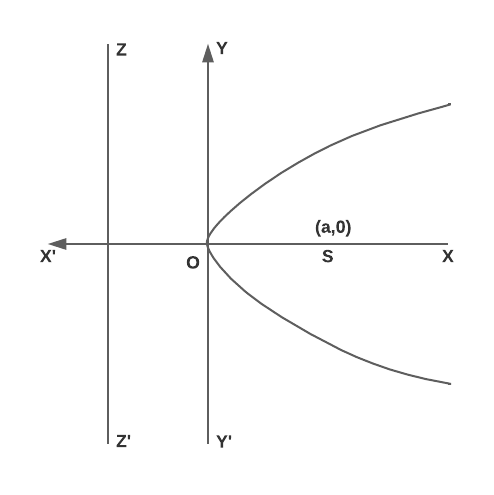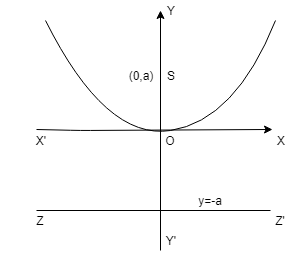# Graph of a Parabola – Types of Parabolas

A parabola is locus of a point which moves in a plane, such that its distance from a fixed point called focus is equal to its perpendicular distance from a fixed straight line called directrix. Graph of a Parabola and their types are shown below.

## Basic Concepts of a Parabola

(a) Focal distance : The distance of a point on parabola from the focus is called focal distance of the point.

(b) Focal chord : A chord of parabola, which passes through focus is called focal chord.

(c) Double ordinate : A chord of the parabola perpendicular to the axis of the symmetry is called double ordinate.

(d) Latus rectum : A double ordinate passing through focus or a focal chord perpendicular to axis of parabola is called latus rectum.

## Types and Graph of a Parabola :

Four standard forms of parabola are $$y^2$$ = 4ax ; $$y^2$$ = -4ax ; $$x^2$$ = 4ay ; $$x^2$$ = -4ay. All of them with their graphs are given below :

(i) Parabola $$y^2 = 4ax$$ :Vertex O is (0, 0)

Focus S is (a, 0)

Axis is y = 0

Directrix ZZ’ is x = -a

Focal length = x + a

Length of Latus rectum = 4a

(ii) Parabola $$y^2 = -4ax$$ :Vertex O is (0,0)

Focus S is (-a,0)

Axis is y = 0

Directrix ZZ’ is x = a

Focal length = x – a

Length of Latus rectum = 4a

(iii) Parabola $$x^2 = 4ay$$ :Vertex O is (0,0)

Focus S is (0,a)

Axis is x = 0

Directrix ZZ’ is y = -a

Focal length = y + a

Length of Latus rectum = 4a

(iv) Parabola $$x^2 = -4ay$$ :Vertex O is (0,0)

Focus S is (0,-a)

Axis is x = 0

Directrix ZZ’ is y = a

Focal length = y – a

Length of Latus rectum = 4a

Hope you learnt basic concepts and graph of a parabola, learn more concepts of parabola and practice more questions to get ahead in the competition. Good luck!